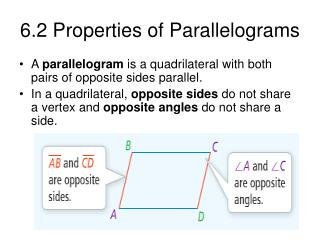DownloadDownload Presentation6.2 Properties of Parallelograms

# 6.2 Properties of Parallelograms

Download Presentation## 6.2 Properties of Parallelograms

- - - - - - - - - - - - - - - - - - - - - - - - - - - E N D - - - - - - - - - - - - - - - - - - - - - - - - - - -
##### Presentation Transcript

1. 6.2 Properties of Parallelograms • A parallelogram is a quadrilateral with both pairs of opposite sides parallel. • In a quadrilateral, opposite sides do not share a vertex and opposite angles do not share a side.

2. Theorem 6.3 • If a quadrilateral is a parallelogram, then its opposite sides are congruent.

3. Consecutive Angles • Angles of a polygon that share a side are consecutive angles.

4. Theorem 6.4 • If a quadrilateral is a parallelogram, then its consecutive angles are supplementary.

5. Using Consecutive Angles • What is the measure of angle P in parallelogram PQRS? • 26° • 64° • 116° • 126°

6. Theorem 6.5 • If a quadrilateral is a parallelogram, then its opposite angles are congruent.

7. Theorem 6.6 • If a quadrilateral is a parallelogram, then its diagonals bisect each other.

8. Using Algebra to Find Lengths • Solve a system of linear equations to find the values of x and y in parallelogram KLMN. What are KM and LN?

9. Using Algebra to Find Lengths

10. Theorem 6.7 • If three (or more) parallel lines cut off congruent segments on one transversal, then they cut off congruent segments on every transversal.

11. Using Parallel Lines and Transversals • In the figure, AE || BF || CG || DH, AB = BC = CD = 2, and EF = 2.25. What is EH? EF = FG = GH EH = EF + FG + GH EH = 2.25 + 2.25 + 2.25 EH = 6.75

12. More Practice!!!!! • Homework – p. 364 - 365 #9 – 12, 14 – 27, 29 – 30 ALL.